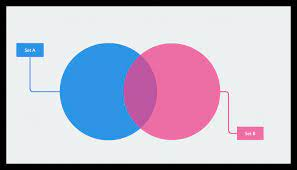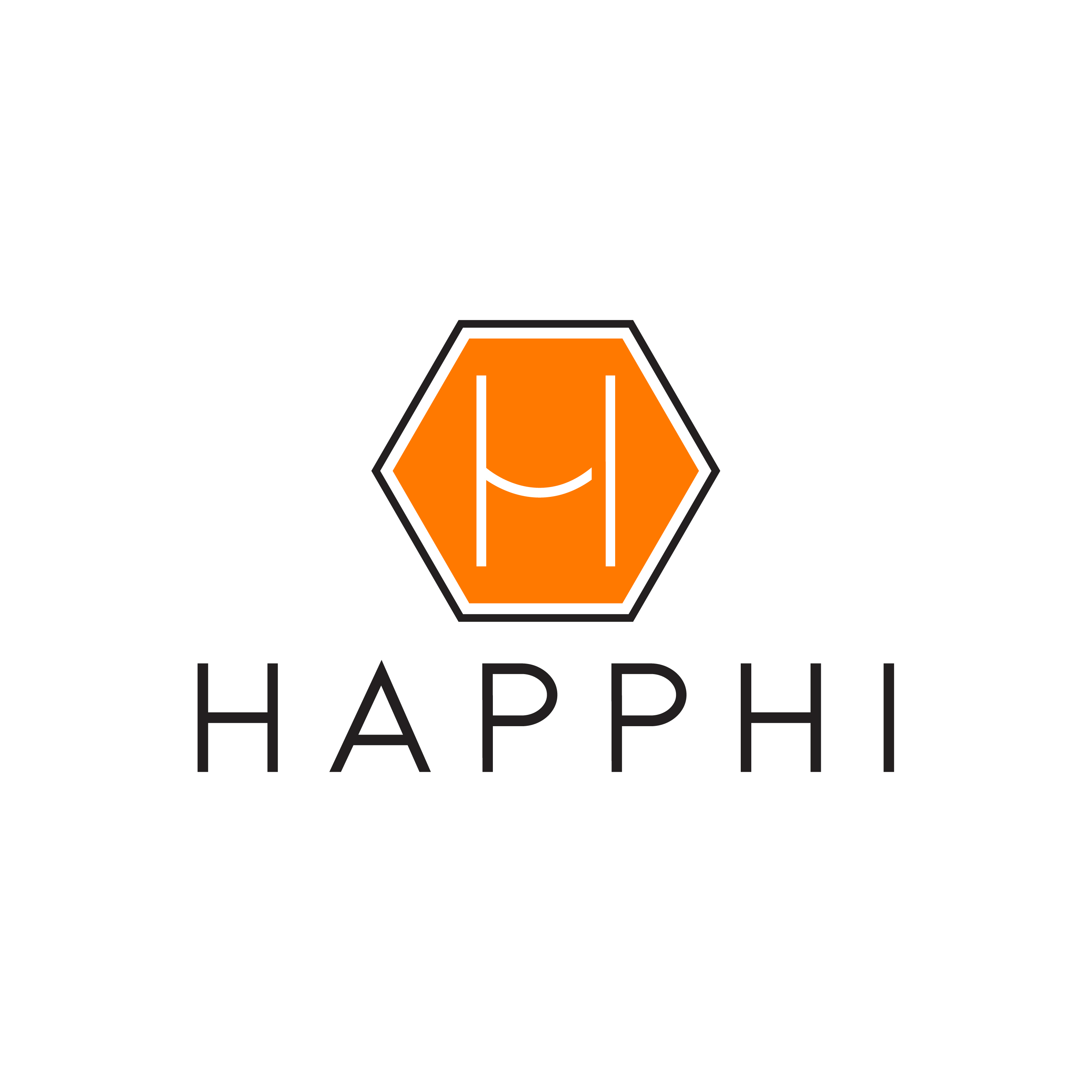Blog
/
No items found.

# Venn Diagram for Compare Search

This post provides a how-to for creating Venn diagrams in HapPhi, with examples and templates. Venn diagrams are used to display the connections between sets of data.Written byJune 15, 2022

## What is a Venn Diagram?

A Venn diagram is a visual tool used to show how two or more things relate to one another. It’s called a “Venn” diagram because the chart is a visualization of the set theory diagrams devised by John Venn in the 1880s. Venn diagrams contain interrelated sets of information, and the overlap of those sets is what allows us to see their relationships. This overlap is often represented by shapes inside the diagram, with each shape corresponding to one of the sets. The shape’s size indicates how large a set is, while its colour and opacity show how closely related it is to the other sets. The diagram below is an example of a basic Venn diagram.

## How to Create a Venn diagram in HapPhi

HapPhi is a diagramming tool that can help you create and share Venn diagrams, flowcharts, and other types of diagrams. You can create a new diagram by going to haphiph.com and clicking on "New Diagram" in the top menu. To create a Venn diagram in HapPhi, follow these steps: - Choose the "Basic" diagram type. - Select either the "Two-set" or "Multi-set" diagram type (see above for the difference). - Choose the orientation of your diagram (horizontal or vertical). - Select the diagram size and colour scheme. - Add your shapes and other elements. - Save the diagram to your computer.

## Venn Diagram Template for comparing 3 things

If you’re comparing three things, use this diagram template. This diagram shows the different sets as circles, with a Venn diagram inside each circle. The overlap in the middle of each Venn diagram represents the shared aspects of each set. - You can add numbers or other text to the circles to label the sets. This can also help you to visually organize your data. - The surrounding rectangle should contain the title of your Venn diagram, along with a legend that labels each set. - Add a visual element to the diagram by inserting a photo or graphic into the Venn diagram in the middle.

## Venn Diagram Template for comparing 2 things

If you’re comparing two things, use this diagram template. This diagram shows the different sets as squares, with a Venn diagram inside each square. The overlap in the middle of each Venn diagram represents the shared aspects of each set. - You can add numbers or other text to the squares to label the sets. - The surrounding rectangle should contain the title of your Venn diagram, along with a legend that labels each set. - Add a visual element to the diagram by inserting a photo or graphic into the Venn diagram in the middle.

## Venn Diagram Template for only 1 thing

If you’re only comparing one thing, use this diagram template. This diagram shows the different sets as a triangle, with a Venn diagram inside each triangle. The overlap in the middle of each Venn diagram represents the shared aspects of each set. - You can add numbers or other text to the triangles to label the sets. - The surrounding rectangle should contain the title of your Venn diagram, along with a legend that labels each set. - Add a visual element to the diagram by inserting a photo or graphic into the Venn diagram in the middle.

## Conclusion

A Venn diagram is a simple way of showing the relationship between different sets of information. This article explains how to create a Venn diagram in Google Sheets, with examples and templates to get you started.

## Get started with HapPhi today

Access all HapPhi features free with 5 free GB, then decide whether you love HapPhi or want to marry HapPhi.

First 1000 people on the list get 100 free tokens.